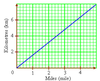# Graphs in practical situation Flashcards Preview

## Maths in Practical Situation II > Graphs in practical situation > Flashcards

Flashcards in Graphs in practical situation Deck (13):
1

2

3

4

## Use the S\$ Euro graph toFind the amount S\$ you get in exchange for Euro\$200### Draw a vertical line from Euro 200 until it touches the line, then draw a horizontal line to determine S\$ amountS\$300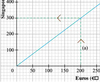5

## Use the S\$ Euro graph toFind the amount Euro\$ you get in exchange for S\$450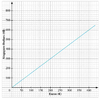### Draw a horizontal line from S\$450 until it touches the line, then draw a vertical line to determine Euro\$ amountEuro\$3006

## Use the S\$ Euro graph toFind the amount Euro\$ you get in exchange for S\$6007

## Use the S\$ Euro graph toFind the amount S\$ you get in exchange for Euro\$100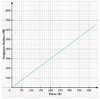8

## Use the Fahrenheit and Celsius graph tofind the value of 50°F in Celsius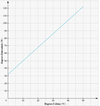### Draw a horizontal line from 50ºF until it touches the line, then draw a vertical line to determine the celsius rate10ºC9

## Use the Fahrenheit and Celsius graph tofind the value of 30°C in Fahrenheit### Draw a vertical line from 30ºC until it touches the line, then draw a horizontal line to determine the Fahrenheit rate85ºF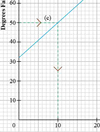10

## Use the Fahrenheit and Celsius graph tofind the value of 20°C in Fahrenheit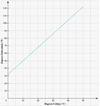11

## Use the Fahrenheit and Celsius graph tofind the value of 70°F in Celsius### Draw a horizontal line from 70ºF until it touches the line, then draw a vertical line to determine the celsius rate21ºC12

## Use the km to mile graph tofind the value of 2 miles in km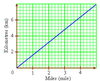13

## Use the km to mile graph tofind the value of 6 km in miles